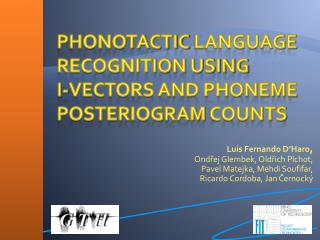DownloadDownload PresentationPhonotactic Language Recognition using i -vectors and Phoneme Posteriogram Counts

# Phonotactic Language Recognition using i -vectors and Phoneme Posteriogram Counts

Download Presentation## Phonotactic Language Recognition using i -vectors and Phoneme Posteriogram Counts

- - - - - - - - - - - - - - - - - - - - - - - - - - - E N D - - - - - - - - - - - - - - - - - - - - - - - - - - -
##### Presentation Transcript

1. Luis Fernando D’Haro, OndřejGlembek, OldřichPlchot, PavelMatejka, MehdiSoufifar, Ricardo Cordoba, Jan Černocký Phonotactic Language Recognition usingi-vectors and Phoneme Posteriogram Counts

2. Content • Introduction • Steps to create Joint-Posteriogram n-gram counts • Subspace Multinomial Models • i-vectors • Results on LRE-2009 • Conclusions and Future work

3. Introduction • Current main approaches to LID • Acoustic-based: i-vectors, JFA, SVMs, or GMMs • Phonotactic • Phonotactic systems: • PRLM, PPRLM: LMs created using different phonetic ASR • Lattice-based soft-counts: Created from phone lattices • Zero counts (i.e. data sparseness) • Limited by the number of phonemes and n-gram order • Dimensionality reduction: PCAor n-gram selection • Using i-vectors through Subspace Multinomial Models (SMMs) • We propose a new feature vector that performs better than soft-counts

4. 1. Compute Posterior Probabilities • In the example, we consider only three phonemes and bigrams. In our experiments, they were 33 and we used trigrams.

5. 2. Compute Posterior Probs • Find the phoneme boundaries using Viterbi algorithm • Can be seen as incorporation of a-priori information • Average the posterior probs over the phone boundaries • Smoothes the posterior probs and avoids the high-correlation of within-phoneme posteriors

6. 3. Create N-gram Posterior Probs • Outer product with the posteriogram of the previous phones • Assume that the frames of the averaged posteriogram are statistically independent, • Therefore we have joint probabilities for sequences of phonemes

7. 4. N-gram Counts via N-gram Posterior Probs • Sum up all matrices to obtain n-gram soft counts • Obtain feature super-vector for creating next the phonotactici-vectors using SMMs

8. Subspace Multinomial Models • Allows extraction of low-dimensional vectors of coordinates in total variability subspace (i.e. i-vectors) • The log-likelihood of data D for a multinomial model with C discrete events is determined by • Where γnc is the count for n-gram event c at utterance n, and φnc is the probability of a multinomial distribution deﬁned by the subspace model

9. i-vectors from SMM • Where tc is the c-th row of subspace matrix T (Extractor), and wn is the i-vector • An i-vector for a single utterance is estimated numerically by maximizing the likelihood (ML) • Matrix T is trained numerically using ML by iteratively optimizing T and re-estimating the i-vectors for all training utterances • Then we use these i-vectors as feature input for training a discriminative LID classifier • Multiclass logistic regression

10. Experimental Setup • NISTLRE 2009 database • 50K segments for training (~119h), 38K segments for dev (~153h) and 31K sentences for test (~125h) • 23 languages, test on 3, 10, and 30 s conditions • Results given using Cavg metric • Acoustic i-vector system • 7 MFCC + 49 SDCs, CMN/CVN, 2048 Gaussians -> i-vectors of 400 dimensions • Comparisons with: • Lattice-based soft-counts with i-vectors (size 600) • Lattice-based soft-counts with PCA (reduction to 1000 dimensions) • Fusion: Multiclass logistic regression • Acoustic and Phonotactic

11. Results on NISTLRE 2009 • 30 s • 10 s • Vertical-axes in logarithm • 3 s

12. Conclusions and Future Work • Advantages of the new features • Avoid data sparseness (i.e. robustness) • Results outperforms a similar system based on lattice soft-counts with i-vectors • 8,16% relative on 30 s condition • Fusion with acoustic i-vectors are also better • 10% relative on 30 s condition • Future Work: Apply discriminative n-gram selection techniques to reduce the vector size • Avoids low frequency n-gram counts • Allows using high n-gram orders